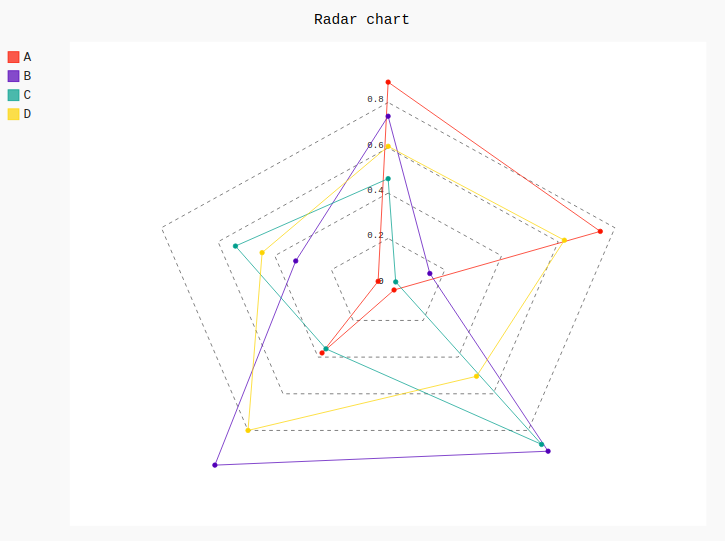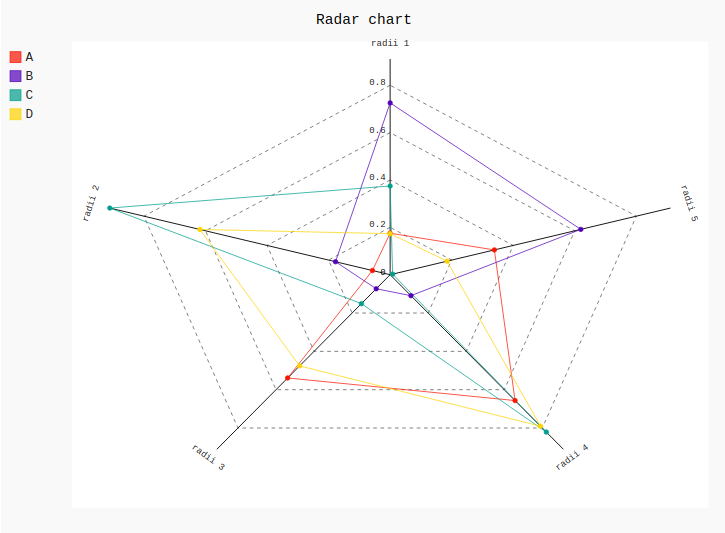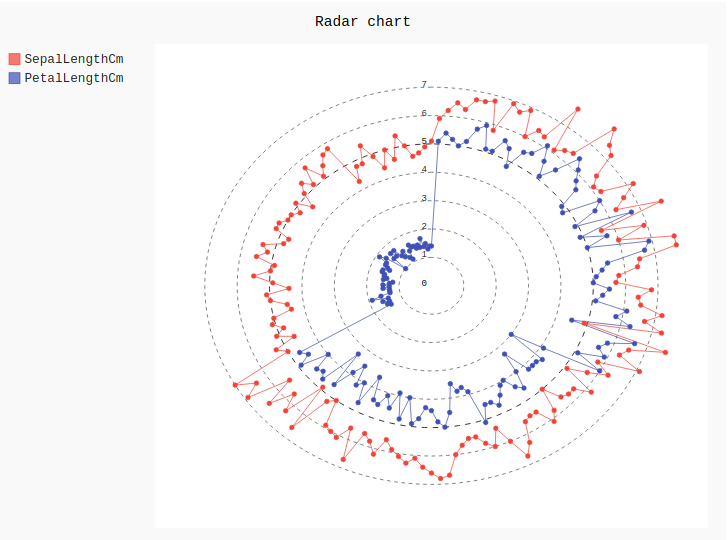Pygal is a Python module that is mainly used to build SVG (Scalar Vector Graphics) graphs and charts. SVG is a vector-based graphics in the XML format that can be edited in any editor. Pygal can create graphs with minimal lines of code that can be easy to understand.

The radar chart is a chart or plot that consists of a sequence of equiangular spokes, called radii, with each spoke representing one of the variables. A radar chart is basically a graphical method of displaying data in the form of a two-dimensional chart of three or more quantitative variables that are represented on the axes starting from the same point. Radar charts are MORE helpful for small-to-moderate-sized multivariate data sets. It can be created using the Radar() method.

Syntax:

```radar_chart = pygal.Radar()
```

Example 1:

## Python3

 `# importing pygal ` `import` `pygal ` `import` `numpy ` ` `  ` `  `# creating the chart object ` `radar_chart ``=` `pygal.Radar() ` ` `  `# naming the title ` `radar_chart.title ``=` `'Radar chart'` ` `  `# Random data ` `radar_chart.add(``'A'``, numpy.random.rand(``5``)) ` `radar_chart.add(``'B'``, numpy.random.rand(``5``)) ` `radar_chart.add(``'C'``, numpy.random.rand(``5``)) ` `radar_chart.add(``'D'``, numpy.random.rand(``5``)) ` ` `  `radar_chart`

Output:Example 2:

## Python3

 `# importing pygal ` `import` `pygal ` `import` `numpy ` ` `  ` `  `# creating the chart object ` `radar_chart ``=` `pygal.Radar() ` ` `  `# naming the title ` `radar_chart.title ``=` `'Radar chart'` ` `  `radar_chart.x_labels ``=` `[``'radii 1'``, ``'radii 2'``, ` `                        ``'radii 3'``, ``'radii 4'``, ` `                        ``'radii 5'``] ` ` `  `# Random data ` `radar_chart.add(``'A'``, numpy.random.rand(``5``)) ` `radar_chart.add(``'B'``, numpy.random.rand(``5``)) ` `radar_chart.add(``'C'``, numpy.random.rand(``5``)) ` `radar_chart.add(``'D'``, numpy.random.rand(``5``)) ` ` `  `radar_chart`

Output:Example 3: Using Iris Dataset

## Python3

 `# importing pygal ` `import` `pygal ` `import` `pandas ` ` `  ` `  `# creating the chart object ` `radar_chart ``=` `pygal.Radar() ` ` `  `# naming the title ` `radar_chart.title ``=` `'Radar chart'` ` `  `df ``=` `pandas.read_csv(``'Iris.csv'``) ` ` `  `radar_chart.add(``"SepalLengthCm"``, df[``'SepalLengthCm'``]) ` `radar_chart.add(``"PetalLengthCm"``, df[``'PetalLengthCm'``]) ` `radar_chart`

Output:My Personal Notes arrow_drop_upIf you like GeeksforGeeks and would like to contribute, you can also write an article using contribute.geeksforgeeks.org or mail your article to contribute@geeksforgeeks.org. See your article appearing on the GeeksforGeeks main page and help other Geeks.

Please Improve this article if you find anything incorrect by clicking on the "Improve Article" button below.

Article Tags :

Be the First to upvote.

Please write to us at contribute@geeksforgeeks.org to report any issue with the above content.